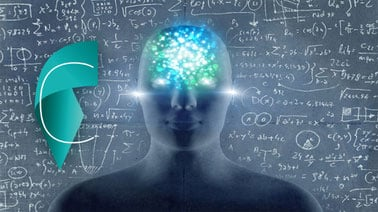# Computational Neuroscience: Neuronal Dynamics of Cognition

This course explains the mathematical and computational models that are used in the field of theoretical neuroscience to analyze the collective dynamics of thousands of interacting neurons.## There is one session available:

After a course session ends, it will be archived.
Estimated 6 weeks
4–6 hours per week
Self-paced
Free

What happens in your brain when you make a decision? And what happens if you recall a memory from your last vacation? Why is our perception of simple objects sometimes strangely distorted? How can millions of neurons in the brain work together without a central control unit?

This course explains the mathematical and computational models that are used in the field of theoretical neuroscience to answer the above questions. The core of the answer to cognition may lie in the collective dynamics of thousands of interacting neurons - and these dynamics are mathematically analyzed in this course using methods such as mean-field theory and non-linear differential equations.

### At a glance

• Institution: EPFLx
• Subject: Biology & Life Sciences
• Prerequisites:

Calculus and Differential equations at the level of a bachelor in physics, math, or electrical engineering.

• Language: English

# What you'll learn

Skip What you'll learn

By the end of the course,you willbe able to:

• Analyze connected networks in the mean-field limit
• Formalize biological facts into mathematical models
• Understand a simple mathematical model of memory formation in the brain
• Understand a simple mathematical model of decision processes
• Understand cortical field models of perception

# Syllabus

Skip Syllabus

Textbook:

Neuronal Dynamics - from single neurons to networks and models of cognition (W. Gerstner, W.M. Kistler, R. Naud and L. Paninski), Cambridge Univ. Press. 2014

online version: http://neuronaldynamics.epfl.ch/

The course will be based on Chapters 12 and 16-19.

Overview of contents over 6 weeks:

A) Associative Memory and Hopfield Model

B) Attractor networks and spiking neurons

C) Neuronal populations and mean-field theory

D) Perception and cortical field models

E) Decision making and competitive dynamics

F) Synaptic Plasticity and learning